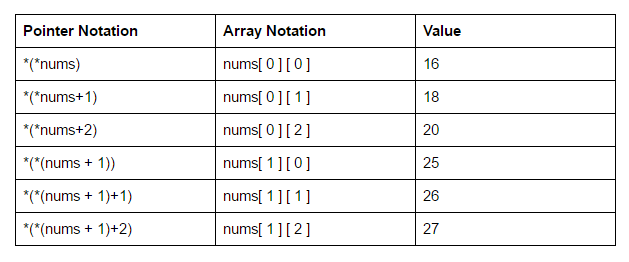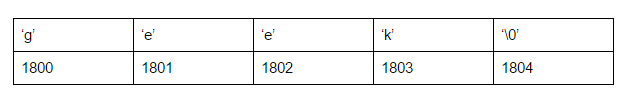# 12.6: Pointer Arithmetic

$$\newcommand{\vecs}{\overset { \rightharpoonup} {\mathbf{#1}} }$$ $$\newcommand{\vecd}{\overset{-\!-\!\rightharpoonup}{\vphantom{a}\smash {#1}}}$$$$\newcommand{\id}{\mathrm{id}}$$ $$\newcommand{\Span}{\mathrm{span}}$$ $$\newcommand{\kernel}{\mathrm{null}\,}$$ $$\newcommand{\range}{\mathrm{range}\,}$$ $$\newcommand{\RealPart}{\mathrm{Re}}$$ $$\newcommand{\ImaginaryPart}{\mathrm{Im}}$$ $$\newcommand{\Argument}{\mathrm{Arg}}$$ $$\newcommand{\norm}{\| #1 \|}$$ $$\newcommand{\inner}{\langle #1, #2 \rangle}$$ $$\newcommand{\Span}{\mathrm{span}}$$ $$\newcommand{\id}{\mathrm{id}}$$ $$\newcommand{\Span}{\mathrm{span}}$$ $$\newcommand{\kernel}{\mathrm{null}\,}$$ $$\newcommand{\range}{\mathrm{range}\,}$$ $$\newcommand{\RealPart}{\mathrm{Re}}$$ $$\newcommand{\ImaginaryPart}{\mathrm{Im}}$$ $$\newcommand{\Argument}{\mathrm{Arg}}$$ $$\newcommand{\norm}{\| #1 \|}$$ $$\newcommand{\inner}{\langle #1, #2 \rangle}$$ $$\newcommand{\Span}{\mathrm{span}}$$$$\newcommand{\AA}{\unicode[.8,0]{x212B}}$$

### Pointer Expressions and Pointer Arithmetic

A limited set of arithmetic operations can be performed on pointers which are:

• incremented ( ++ )
• decremented ( -- )
• an integer may be added to a pointer ( + or += )
• an integer may be subtracted from a pointer ( – or -= )
• difference between two pointers (p1-p2)

(Note: Pointer arithmetic is meaningless unless performed on an array.)

// C++ program to illustrate Pointer Arithmetic in C++
#include <bits/stdc++.h>
using namespace std;
int main()
{
//Declare an integer array
int intArr = {10, 100, 200};

//declare an integer pointer variable
int *arrPtr;

//Assign the address of intArr to arrPtr
arrPtr = intArr;

for (int count = 0; count < 3; count++)
{
cout << "Value at arrPtr = " << arrPtr << "\n";
cout << "Value at *arrPtr = " << *arrPtr << "\n";

// Increment pointer ptr by 1
arrPtr++;
}
return 0;
}


(Note: Pointer arithmetic is meaningless unless performed on an array.)

In the code above we increment the pointer by 1. Look at the output of the addresses below - each address is 4 greater than the previous address!! How is that, we only added one, using the ++ operator, to the pointer variable?

Output:
Value at arrPtr = 0x7fff9a9e7920
Value at *arrPtr = 10
Value at arrPtr = 0x7fff9a9e7924
Value at *arrPtr = 100
Value at arrPtr = 0x7fff9a9e7928
Value at *arrPtr = 200


C++ knows that the variable - both the array and the pointer - are an integer type, so adding one to that address means we move forward the width of one integer, which is usually 4 bytes.

The subscript - that value in the [ ] - says to go a certain number of bytes past that address. So, the first element , says to go 0 bytes beyond the address. When we use  - C++ says, "okay, this is an integer (in our example), so I will go 1 integer width past the address in intArr. An integer, on this system, is 4 bytes long, so intArr look 4 bytes past the address stored in intArr.

Consider pointer notation for the two-dimensional numeric arrays. consider the following declaration

int nums  =  { { 16, 18, 20 }, { 25, 26, 27 } };

In general, nums[ i ][ j ] is equivalent to *(*(nums+i)+j)### Pointers and String literals

String literals are arrays containing null-terminated character sequences. String literals are arrays of type character plus terminating null-character, with each of the elements being of type const char (as characters of string can’t be modified).

const char * ptr = "geek";

This declares an array with the literal representation for “geek”, and then a pointer to its first element is assigned to ptr. If we imagine that “geek” is stored at the memory locations that start at address 1800, we can represent the previous declaration as:As pointers and arrays behave in the same way in expressions, ptr can be used to access the characters of string literal. For example:

char x = *(ptr+3);
char y = ptr;

Here, both x and y contain k stored at 1803 (1800+3).